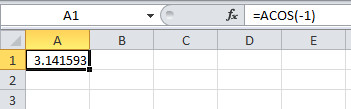# ACOS Function In Excel To Return Inverse Cosine

In this tutorial, learn how to use ACOS function in Excel to find the inverse of cosine of a number. It returns an angle which you have to convert in degree using the below methods.

## What is the ACOS Function in Excel

An ACOS function is the trigonometric function under the category Math/Trig of Excel. It is a built-in function of MS Excel you can use when you want to calculate the inverse of cosine.You can find the inverse cosine angle with `ACOS()` using the methods given below.

## Syntax of ACOS Function

The syntax of the ACOS function is given below which contains the single argument.

## Argument Passing to ACOS Function

There is only a single argument or parameter of the function to pass. See the description of the argument is given below.

Parameter Name Description
number The number is the value between -1 to 1 which you have pass as argument.

Now, let’s check the various examples to find the inverse cosine of a number.

## Example to Find Inverse Cosine of a Number

There are many examples given below to find the inverse cosine of value from -1 to 1.

Example 1

Output

1.047198

Example 2The -1 number if pass as the argument will give the value if PI() as the output. The value of the PI is 3.141593 as given in the below example.

Output

3.141593

Example 3

Output

0

SO, you have calculated the inverse cosine of any number between -1 to 1. The above outputs are in radian and not in degree. To convert them to a degree, you have to follow the method given in the next section. So, read further to find the requires method.

## How to Convert Radian to Degree

To convert radian to a degree, you have to multiply the inverse cosine value with the value `180/PI()`. Check the below example to use and convert your output to a degree.

Example 1

Output

60

Example 2Output

180

Example 3

Output

0

Hope, you like this post of how to use ACOS function in Excel. If you have any query regarding the tutorial, please comment below.

Also tell me, which method you are using to get the inverse cosine of a number in Microsoft Excel.

Reference
MS Office Support